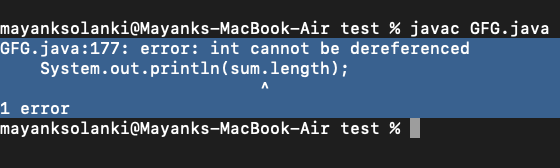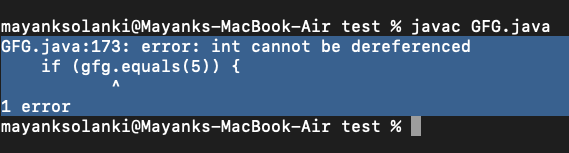# How to Fix int cannot be dereferenced Error in Java?

• Difficulty Level : Medium
• Last Updated : 21 Jul, 2021

Here, we will follow the below-mentioned points to understand and eradicate the error alongside checking the outputs with minor tweaks in our sample code

• Introduction about the error with example
• Explanation of deferencing in detail
• Finally, how to fix the issue with Example code and output.

Attention reader! Don’t stop learning now. Get hold of all the important Java Foundation and Collections concepts with the Fundamentals of Java and Java Collections Course at a student-friendly price and become industry ready. To complete your preparation from learning a language to DS Algo and many more,  please refer Complete Interview Preparation Course.

Example 1:

## Java

 `// Java Program to Illustrate Dereference` `// Importing required classes``import` `java.io.*;``import` `java.util.*;` `// Main class``class` `GFG {` `    ``// Main driver method``    ``public` `static` `void` `main(String[] args)``    ``{``        ``// Reading input from keyboard by``        ``// creating object of Scanner class``        ``Scanner sc = ``new` `Scanner(System.in);` `        ``// Taking input for two integer variables``        ``int` `a = sc.nextInt();``        ``int` `b = sc.nextInt();` `        ``// Calculating sum of two variables and storing the``        ``// value in sum variable``        ``int` `sum = a + b;` `        ``//  Print and display resultant length``        ``System.out.println(sum.length);``    ``}``}`

Output:So this is the error that occurs when we try to dereference a primitive. Wait hold on what is deference now?. Let us do talk about that in detail. In Java there are two different variables are there:

1. Primitive [byte, char, short, int, long, float, double, boolean]
2. Objects

Since primitives are not objects so they actually do not have any member variables/ methods. So one cannot do Primitive.something(). As we can see in the example mentioned above is an integer(int), which is a primitive type, and hence it cannot be dereferenced. This means sum.something() is an INVALID Syntax in Java.

Explanation of Java Dereference and Reference:

• Reference: A reference is an address of a variable and which doesn’t hold the direct value hence it is compact. It is used to improve the performance whenever we use large types of data structures to save memory because what happens is we do not give a value directly instead we pass to a reference to the method.
• Deference: So Deference actually returns an original value when a reference is Dereferenced.

What deference Actually is?

Deference actually means we access an object from heap memory using a suitable variable. The main theme of Deferencing is placing the memory address into the reference. Now, let us move to the solution for this error,

How to Fix “int cannot be dereferenced” error?

Note: Before moving to this, to fix the issue in Example 1 we can print,

`System.out.println(sum); // instead of sum.length  `

Calling equals() method on the int primitive, we encounter this error usually when we try to use the .equals() method instead of “==” to check the equality.

Example 2:

## Java

 `// Main class``public` `class` `GFG {` `    ``// Main driver method``    ``public` `static` `void` `main(String[] args)``    ``{` `        ``// Declaring and initialising the variable``        ``// with integer value``        ``int` `gfg = ``5``;` `        ``// Checking the equality using .equals() method``        ``if` `(gfg.equals(``5``)) {` `            ``// Print statement``            ``System.out.println(``"The value of gfg is 5"``);``        ``}``        ``else` `{` `            ``// Print statement``            ``System.out.println(``"The value of gfg is not 5"``);``        ``}``    ``}``}`

Output:Still, the problem is not fixed. We can fix this issue just by replacing the .equals() method with”==” so let’s implement “==” symbol and try to compile our code.

Example 3:

## Java

 `public` `class` `EqualityCheck {` `    ``public` `static` `void` `main(String[] args)``    ``{` `        ``int` `gfg = ``5``;``        ``// Instead of .equals method here we have used "=="``        ``// symbol to check the equality``        ``if` `(gfg == ``5``)` `        ``{` `           ``System.out.println(``"The value of gfg is 5"``);``        ``}` `        ``else` `        ``{` `           ``System.out.println(``"The value of gfg is not 5"``);``        ``}``    ``}``}`
Output
`The value of gfg is 5`

This is it, how to fix the “int cannot be deferenced error in Java.

My Personal Notes arrow_drop_up